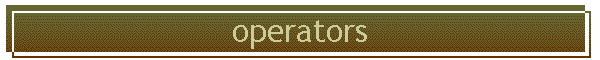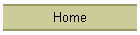## modulus operator %

The % operator is the same as the Fortran mod function, returning the remainder after integer division.

This provides an easy way to test whether an integer variable is even or odd:
if (!(number%2))
will evaluate as false (0) if number is even, and true (1) if odd.

C provides an even faster way to do this - test whether the lowest bit is 0 or 1:
if ( number & 1)
/*  odd  */
else
/*  even */

## the sizeof operator

This takes a variable or array as an argument, and returns the number of bytes it contains.  For instance, to obtain enough space for a double precision array of length of 10, one would call
malloc(10*sizeof(double));

## prefix and postfix operations . . .

Performing an operation on a variable, and saving the result in the same variable, happens so often that C has a shorthand for it.  The following pairs of statements are equivalent:

i = i + 1;
i += 1;
i =+ ;
i++;
++i;

j = j + 2;
j += 2;
j =+ 2;

a = a / 2.;
a /= 2.;
a =/ 2.;

There is no reason to use this, except for brevity.

## and side effects

Side effects are when a single line does more than one thing.  They are a source of pride for people who code too much C, but are avoided by the rest of us, who want to write clear code.  As an example, consider
long i , k;
i = 8;
k = ++i;
The last statement sets both k and i to 9.  Had the last statement been reverse,
k = i++;
we would have k = 8 and i =9.  The difference is the difference between prefix vs postfix operators.  A prefix operator ( ++i, +=, /=) does the operation to the right hand variable before assigning it to the left hand, and a postfix operator (i++,+,/) does the operation after.  If side effects are not used, so there is one operation per line, there will be no difference.

## Relational operators

 operator meaning <= less than or equal < less than > greater than >= greater than or equal == equal != not equal

## logical operators

 operator meaning examples << shift left 1 bit 010 = << 001 >> shift right 1 bit 010 = >> 100 & and 0=0&0; 0=0&1; 1=1&1; | or 0=0|0; 1=1|0; ^ exclusive or (xor) 0=0^0; 1=1^0; 0=1^1; ~ ones complement operator (not) 1=~0; 0=~1;

## exponentiation

Fortran has the a**b function, which raises a to the power b.  C does not support this as a fundamental form of operation.  Rather, math.h includes the pow function, which would be coded as pow(a,b).